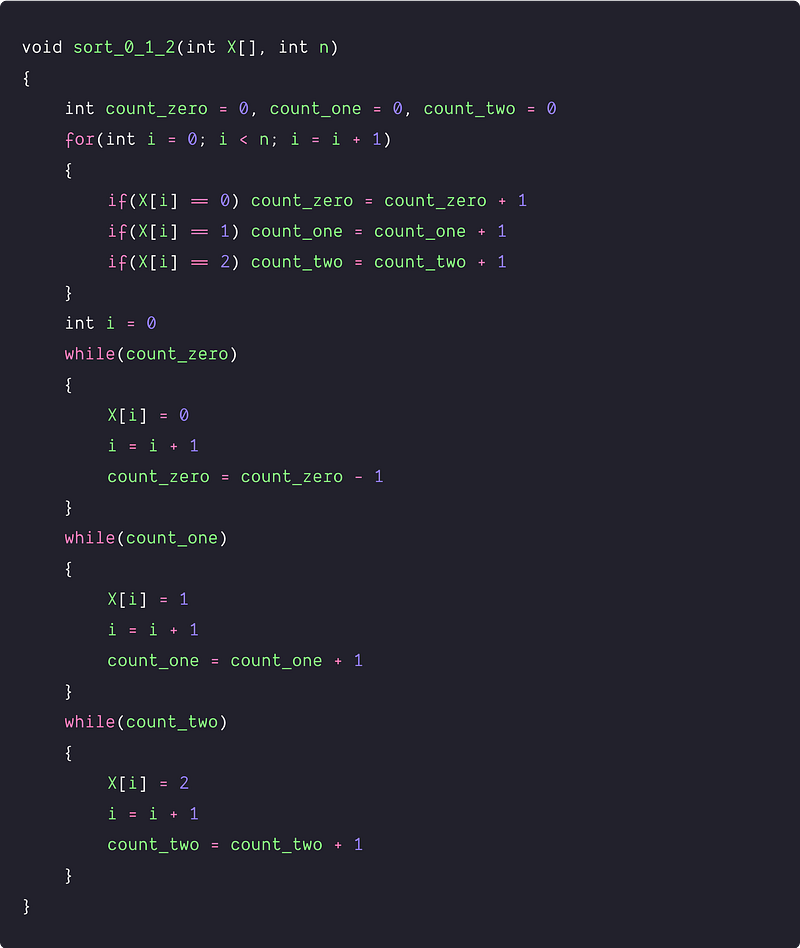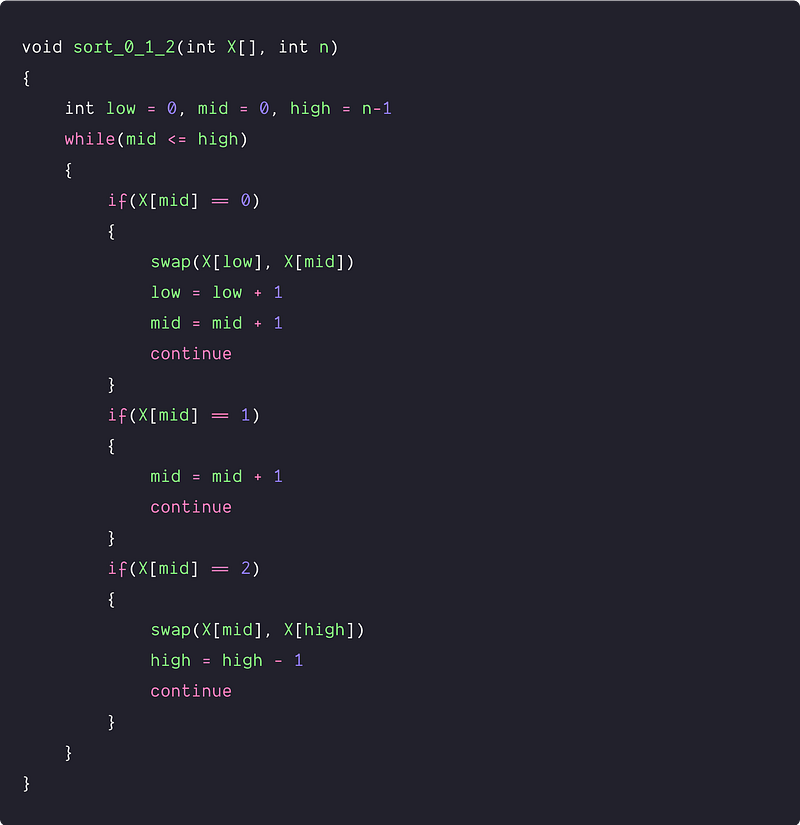# Sort an array of 0s, 1s, and 2s —Dutch national flag problem

Key takeaway

• A special sorting problem that can be solved using O(n) time complexity. There is a lot of variation available to this coding problem.
• For the efficient solution, we are using a single loop and three-pointers to build the partial solution. This problem is a simple variation of the famous Dutch national flag problem.

### Let’s understand the problem

Given an array X[] consisting of 0s, 1s, and 2s, write a program to sort the array of 0’s, 1’s, and 2’s in ascending order.

#### Examples

Input: X[] = [0, 2, 1, 0, 1, 2, 1, 0], Output: X[] = [0, 0, 0, 1, 1, 1, 2, 2]

Input: X[]= [0, 1, 1, 0, 1, 2, 1, 2, 0, 0], Output: X[] = [0, 0, 0, 0, 1, 1, 1, 1, 2, 2]

Input: X[] = [2, 1, 0], Output: X[] = [0, 1, 2]

### Discussed solution approaches

1. A brute force approach using double traversal
2. An efficient approach using single scan: Three-way partitioning

### A brute force approach using double traversal

Solution Idea and Steps

The first approach that comes to mind is to simply count the number of 0’s, 1’s, and 2’s. Then, place all 0’s at the beginning of the array followed by 1’s and 2's.

1. Declare three variables countzero, countone, count_two to store the respective frequency of appearances of 0’s, 1’s, and 2’s in the array.
2. Traverse through the array and update the frequency of 0’s, 1’s, and 2's.
3. Now again, traverse the array and replace first countzero places of the array as 0, next countone by 1, and last count_two by 2.

Solution PseudocodeTime and space complexity analysis

Time complexity = Time complexity of counting the number of 0’s, 1’s, and 2’s + Time complexity of placing all 0’s, 1’s, and 2’s in the array = O(n) + O(n) = O(n). As no extra space is required, the space complexity of the above code is O(1).

### An efficient approach using single scan: Three-way partitioning

Solution Idea

We can solve the problem using a single scan by maintaining the correct order of 0’s, 1’s, and 2’s using variables. Actually, we have three types of elements to be placed in sorted order, therefore, we divide the given array into four sections using three-pointers. Let us name these pointers as low, mid, and high.

1. X[0…low-1] only zeroes
2. X[low..mid-1] only ones
3. X[mid…high] unknown
4. X[high+1..n-1] only twos
5. Think of low and high as the range [low, high) for values in the middle partition. The middle partition will contain the values from low up to, but not including, high.
6. All values are less than low end up in the left partition.
7. Finally, all values greater than or equal to high end up in the right partition.

Solution Steps

• Initialise three pointers low = 0, mid = 0 and high = n -1

Run a loop until mid<=high

• If (X[mid] ==0), then swap the element with the element low and shrink the unknown range by doing low++ and mid++.
• If (X[mid] ==1), then simply increment mid (mid++), thus further shrinking unknown range.
• If (X[mid] ==2), then swap it with an element in the high range and decrement high (high--). Then, again traverse the array from mid-index only, as the element at this index has not been considered yet.

Solution PseudocodeTime and space complexity analysis

In the worst case, we are traversing and accessing each element of the array only once. Time complexity = O(n). Space complexity = O(1), As we are using a constant number of variables.

### Critical ideas to think!

• Can we optimize the above code and reduce the swap operations?
• Is this algorithm stable? If not, then how to make it stable?
• Why are we not increasing the mid-index if (X[mid] ==2)?
• Can we solve the problem using the partition process of the quick sort?
• Can we solve this problem using two-pointers?
• How can we modify the above code for a similar problem to sort the array containing 0s,1s, 2s, and 3s?
• What are the famous linear time sorting algorithms? Can we apply the idea of counting sort to solve the above problem?

### Comparisons of time and space complexities

• Brute force approach: Time = O(n), Space = O(1)
• Three-way partitioning: Time = O(n), Space = O(1)

### Suggested coding questions to practice

Thanks to Navtosh Kumar for his contribution in creating the first draft. Please write comments if you find an error or you want to share more insights about the topic. Enjoy learning, Enjoy coding, Enjoy algorithms!

Get well-designed application and interview centirc content on ds-algorithms, machine learning, system design and oops. Content will be delivered weekly.